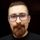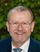## Allow PROC SORT to output multiple datasets

Frequently a SAS programmer needs to both sort and divide a dataset. Typically these tasks are satisfied via a two stage program:   (1) divide the dataset, (2) sort the parts. Something like:

``````data  _asia (where=(origin='Asia'))  cars_europe (where=(origin='Europe'))
cars_usa (where=(origin='USA')) /view=_asia;
set sashelp.cars;
run;
proc sort data=_asia out=cars_asia;
by msrp;
run;
proc sort data=cars_europe;
by msrp;
run;
proc sort data=cars_usa;
by msrp;
run;
``````

Enabling proc sort to do both of these tasks (with syntax such as below) would often significantly reduce input/output activity, and offer a bit of code simplification:

``````proc sort data=sashelp.cars
out=cars_asia   (where=(origin='Asia'))
cars_europe (where=(origin='Europe'))
cars_usa    (where=(origin='USA'))    ;
by msrp;
run;``````

Of course, the multiple output datasets need not be mutually exclusive like the example above.Meteorite | Level 14

Mark, two things:

1) I like the idea you have my voice on this one!

2) For "code simplification" case, you can always do one sorting:

``````proc sort data=sashelp.cars out=cars_sorted;
by msrp;
run;
data  cars_asia   (where=(origin='Asia'))
cars_europe (where=(origin='Europe'))
cars_usa    (where=(origin='USA'))
;
set cars_sorted;
run;``````

All the best

BartSuper User

The syntactically correct way would be to not have multiple datasets in the OUT= option, but to allow multiple OUT= options.Meteorite | Level 14

It would be also nice to make the KEY statement finally work better than "documented" 😉

`Specifies sorting keys and variables. The KEY statement is an alternative to the BY statement. The KEY statement syntax allows for the future possibility of specifying different collation options for each KEY variable. Currently, the only options allowed are ASCENDING and DESCENDING.`

BartMeteorite | Level 14

Kurt, or maybe:

``OUT = ( dataSet1 dataSet2 ... )``

?

BartSuper User

@yabwon on second thought, I think the brackets are better than the multiple options.I had considered using the sort first/split second code

``````proc sort data=sashelp.cars out=cars_sorted;
by msrp;
run;
data  cars_asia   (where=(origin='Asia'))
cars_europe (where=(origin='Europe'))
cars_usa    (where=(origin='USA'))
;
set cars_sorted;
run;``````

as the foil to contrast to my proposal.  But since my primary justification was for efficiency, I chose to start out with the most efficient currently available technique - split first (using a view for one of the splits), and sort second.As to optimal syntax (multiple out= vs multiple arguments of a single out=), I used the data statement as my model for the out= syntax.   I don't have a strong feeling on this issue, as long as it seamlessly supports the use of current dataset name options, especially (of course) where=, keep=, drop=, rename=.SAS Employee
Status changed to: Under Consideration

I've forwarded this request to our Product Management team  - thanks!Great!SAS Employee

With this following syntax, which looks almost identical to that shown by @yabwon you can accomplish the goal here, which is to avoid the extra IO from reading the sorted file to subsequently split into multiple datasets.  This uses the experimental feature called "Output Data Step Views" which are incredibly powerful!

``````data cars_asia(where=(origin='Asia'))
cars_europe(where=(origin='Europe'))
cars_usa(where=(origin='USA'))
/
view=split_sort;

if 0 then set sashelp.cars;
set split_sort;
run;

proc sort data=sashelp.cars out=split_sort;
by msrp;
run;``````
```1   data cars_asia(where=(origin='Asia'))
2        cars_europe(where=(origin='Europe'))
3        cars_usa(where=(origin='USA'))
4        /
5        view=split_sort;
6
7     if 0 then set sashelp.cars;
8     set split_sort;
9   run;
NOTE: DATA STEP view saved on file WORK.SPLIT_SORT.
NOTE: A stored DATA STEP view cannot run under a different operating system.
WARNING: The definition of an output DATA step view is an experimental feature in this release and is not intended for use in the
development of production applications.

10
11   proc sort data=sashelp.cars out=split_sort;
12     by msrp;
13   run;
NOTE: There were 428 observations read from the data set SASHELP.CARS.
NOTE: The data set WORK.SPLIT_SORT has 428 observations and 15 variables.
NOTE: The data set WORK.CARS_ASIA has 158 observations and 15 variables.
NOTE: The data set WORK.CARS_EUROPE has 123 observations and 15 variables.
NOTE: The data set WORK.CARS_USA has 147 observations and 15 variables.```